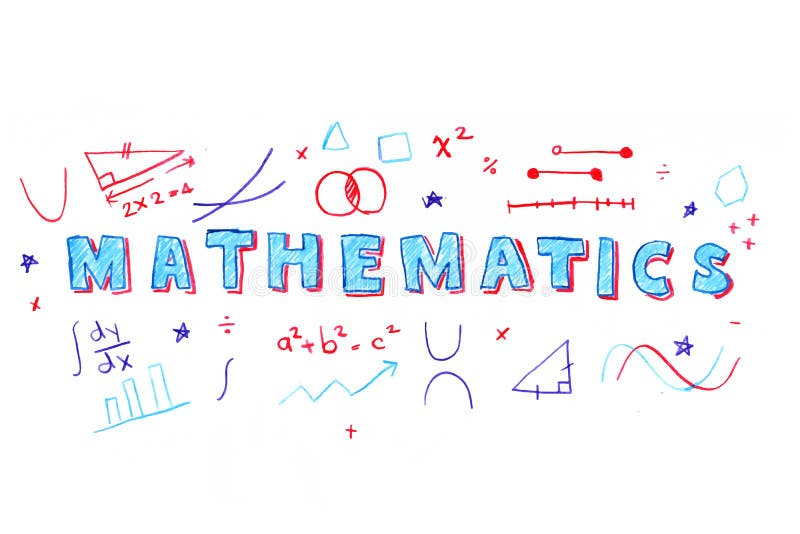•
•
•
•
•
•

#MA8251 ENGINEERING MATHEMATICS – II NOTES 2017 REGULATION

In this post we have posted some notes on MA8251 ENGINEERING MATHEMATICS – II of BASIC SCIENCES for ANNA UNIVERSITY AFFILIATED COLLEGES STUDENTS.
Here We have listed the the notes whichever we could collect for MA8251 ENGINEERING MATHEMATICS – II subject, We think that it will be helpful for your exam preparation.

#### OBJECTIVES:

This course is designed to cover topics such as Matrix Algebra, Vector Calculus, Complex
Analysis and Laplace Transform. Matrix Algebra is one of the powerful tools to handle practical problems arising in the field of engineering. Vector calculus can be widely used for modelling the various laws of physics. The various methods of complex analysis and Laplace transforms can be used for efficiently solving the problems that occur in various branches of engineering disciplines.

#### UNIT I MATRICES

Eigenvalues and Eigenvectors of a real matrix – Characteristic equation – Properties of Eigenvalues and Eigenvectors – Cayley-Hamilton theorem – Diagonalization of matrices – Reduction of a quadratic form to canonical form by orthogonal transformation – Nature of quadratic forms.

#### UNIT II VECTOR CALCULUS

Gradient and directional derivative – Divergence and curl – Vector identities – Irrotational and Solenoidal vector fields – Line integral over a plane curve – Surface integral – Area of a curved surface – Volume integral – Green‘s, Gauss divergence and Stoke‘s theorems – Verification and application in evaluating line, surface and volume integrals.

#### UNIT III ANALYTIC FUNCTIONS

Analytic functions – Necessary and sufficient conditions for analyticity in Cartesian and polar
coordinates – Properties – Harmonic conjugates – Construction of analytic function – Conformal
mapping – Mapping by functions
1 2 , z , z, w, z, c, cz, , – Bilinear transformation.

#### UNIT IV COMPLEX INTEGRATION

Line integral – Cauchy‘s integral theorem – Cauchy‘s integral formula – Taylor‘s and Laurent‘s series – Singularities – Residues – Residue theorem – Application of residue theorem for evaluation of real integrals – Use of circular contour and semicircular contour.

#### UNIT V LAPLACE TRANSFORMS

Existence conditions – Transforms of elementary functions – Transform of unit step function and unit impulse function – Basic properties – Shifting theorems -Transforms of derivatives and integrals – Initial and final value theorems – Inverse transforms – Convolution theorem – Transform of periodic functions – Application to solution of linear second order ordinary differential equations with constant coefficients.

#### OUTCOMES :

After successfully completing the course, the student will have a good understanding of the following topics and their applications:
👉 Eigen values and eigenvectors, diagonalization of a matrix, Symmetric matrices, Positive
definite matrices and similar matrices.
👉 Gradient, divergence and curl of a vector point function and related identities.
👉 Evaluation of line, surface and volume integrals using Gauss, Stokes and Green‘s
theorems and their verification.
👉 Analytic functions, conformal mapping and complex integration.
👉 Laplace transform and inverse transform of simple functions, properties, various related theorems and application to differential equations with constant coefficients.

#### TEXT BOOKS:

1. Grewal B.S., ―Higher Engineering Mathematics‖, Khanna Publishers, New Delhi, 43rd Edition, 2014.
2. Kreyszig Erwin, “Advanced Engineering Mathematics “, John Wiley and Sons,
10th Edition, New Delhi, 2016.

#### REFERENCES :

1. Bali N., Goyal M. and Watkins C., ―Advanced Engineering Mathematics‖, Firewall Media (An imprint of Lakshmi Publications Pvt., Ltd.,), New Delhi, 7th Edition, 2009.
2. Jain R.K. and Iyengar S.R.K., ― Advanced Engineering Mathematics ‖, Narosa
Publications, New Delhi , 3rd Edition, 2007.
3. O‘Neil, P.V. ―Advanced Engineering Mathematics‖, Cengage Learning India
Pvt., Ltd, New Delhi, 2007.
4. Sastry, S.S, ―Engineering Mathematics”, Vol. I & II, PHI Learning Pvt. Ltd, 4 th Edition, New Delhi, 2014.
5. Wylie, R.C. and Barrett, L.C., ―Advanced Engineering Mathematics ―Tata McGraw Hill Education Pvt. Ltd, 6th Edition, New Delhi, 2012.

#### MA8251 ENGINEERING MATHEMATICS – II NOTES

If you have any queries or comments on this post, kindly contact us through contact form or telegram